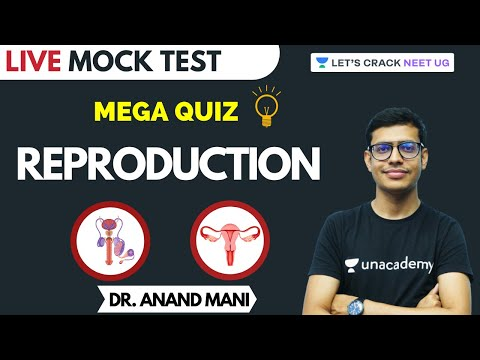Courses

# JIPMER Aptitude Mock Test 5

## 10 Questions MCQ Test JIPMER: Subject wise Tests & Practice Mock Tests | JIPMER Aptitude Mock Test 5

Description
This mock test of JIPMER Aptitude Mock Test 5 for NEET helps you for every NEET entrance exam. This contains 10 Multiple Choice Questions for NEET JIPMER Aptitude Mock Test 5 (mcq) to study with solutions a complete question bank. The solved questions answers in this JIPMER Aptitude Mock Test 5 quiz give you a good mix of easy questions and tough questions. NEET students definitely take this JIPMER Aptitude Mock Test 5 exercise for a better result in the exam. You can find other JIPMER Aptitude Mock Test 5 extra questions, long questions & short questions for NEET on EduRev as well by searching above.
QUESTION: 1

### Four girls A, B, C and D are sitting in a circle. B and C are facing each other. Which of the following is definitely true?

Solution: In question mention it A,B,C and D sitting in a circle and A & C are facing each other and if we are supposed then confused about direction because it is not mentioned (direction) clockwise or anticlockwise then we are supposed all 4 members is sitting in circle on 90′ then correct answer is C
QUESTION: 2

Solution:
QUESTION: 3

### Ramu is sixth from the left end and Hari is tenth from the right end in a row of boys. If there are eight boys between Ramu and Hari, how many boys are there in the row?

Solution:
QUESTION: 4

Arrange the following in a meaningful order:
1. Country
2. Furniture
3. Forest
4. Wood
5. Trees

Solution:
QUESTION: 5

If A means 'x', B means '÷', C means '-' and D means '+' then -
4 D 16 A 5 B 8 C 5 = ?

Solution:

4 D 16 A 5 B 8 C 5
= 4 + 16 x 5 ÷ 8 - 5 (Use BODMAS Rule)
= 4 + 10 - 5
= 9

QUESTION: 6

Choose the correct alternative that will continue the same pattern and fill in the blank
15, __, 27, 27, 39, 39

Solution:

In this simple addition with repetition series, each number in the series repeats itself, and then increases by 12 to arrive at the next number.

QUESTION: 7

Complete the analogous pair.
211 : 333 :: 356 : ?

Solution:

Addition of sum of digits of 2nd term (333) and 3rd term (356) = 9 + 14 = 23
Again addition of sum of digits of 1st term (211) and option (A)(358) = 4 + 16 ≠ 23
Again addition of sum of digits of 1st term (211) and option (B)(329) = 4 + 14 ≠ 23
Again addition of sum of digits of 1st term (211) and option (C)(423) = 4 + 9 ≠ 23
Again addition of sum of digits of 1st term (211) and option (D)(388) = 4 + 19 = 23

QUESTION: 8

In the number 76534218 each digit is replaced by the next digit ie '1' is replaced by '2', '2' is replaced by '3' and so on and then the digits are arranged in ascending order from left to right, which digit will be fifth from the left end?

Solution: According to the rule 76534218~87645329.if we arrange them in ascending order from left then it will be 98765432.the digit at 5th place is 6 from left.
QUESTION: 9

Below is given statement followed by three conclusions numbered I, II and III. You have to consider the statement and the following conclusions and decide which of the conclusions is follows in the statement :
Statements :
a. Some chairs are flowers.
b. All flowers are trees.
c. Some trees are leaves.
Conclusions:I. Some trees are chairs.
II. Some leaves are flowers.
III. No chair is a leaf.

Solution:
QUESTION: 10

Below are the statements followed by three conclusions numbered I, II and III. You have to consider the statements and the following conclusions and decide which of the conclusion(s) follows the statement(s).

Statements :
Conclusions :
II. Some stones are trees.
III. No stone is tree.

Solution:

Track your progress, build streaks, highlight & save important lessons and more!

### Similar Content### Related tests